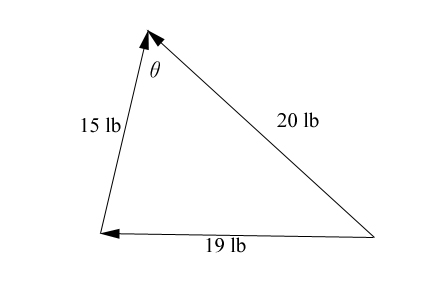SEARCH HOMEMath Central Quandaries & QueriesQuestion from lauren, a student: once force of 20 pounds and one force of 15 pounds act on a body at the same point so that the resultant force is 19 pounds. Find, to the nearest degree, the angle between the two original forcesHi Lauren,

I beleive this is the diagram that goes with the situation you are describing.The easiest way to solve for θ, the angle between the original two vectors, is to use the Law of Cosine.

Hope this helps,

JaniceMath Central is supported by the University of Regina and The Pacific Institute for the Mathematical Sciences.